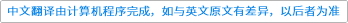Mathematical Sciences ( IF 0.945 ) Pub Date : 2021-06-10 , DOI: 10.1007/s40096-021-00414-4
Yaser Rostami

This article gives a numerical solution for solving the two-dimensional nonlinear Fredholm–Volterra partial integro-differential equations with boundary conditions with weakly singular kernels. The collocation method has been used for these operational matrices of the Taylor wavelet along with the Newton method to reduce the given partial integro-differential equation to the system of algebraic equations. Error analysis is considered to indicate the convergence of the approximation used in this method. Attaining this purpose, first, two-dimensional Taylor wavelet and then operational matrices should be defined. Regarding the characteristics of the Taylor wavelet, we were obtaining high accuracy of the method. Finally, examples are provided to demonstrate that the proposed method is effective.down
wechat
bug